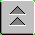Introduction to Naval Weapons Engineering

Electro-Optical Imaging Systems

The Field of View (FOV)

The field-of-view (FOV) is the range of angles from which the incident radiation can be collected by the detector. The field of view may be decomposed into its horizontal and vertical components, labeled as HFOV and VFOV respectively. In both cases the FOV is determined by a combination of the focal length of the lens, f, and the size of the field stop, DF.S..

The focal length of a lens is the distance from the center of the lens to the point where all of the incident radiation (or light) coming from a source at infinity will be focused. If the source is at infinity (or very far away), the incident rays of radiation will be nearly parallel. The lens will refract them all to the same point, namely the focal point of the lens.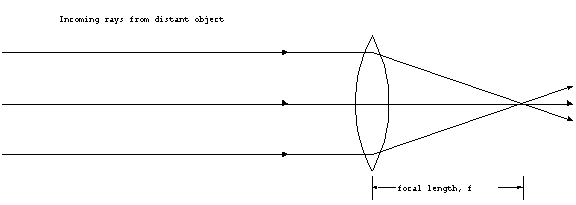Figure 1. Focal point
of a lens.

The field stop is a device that blocks rays that are beyond its dimensions from reaching the detecting element(s). The detecting elements are located at the focal plane, which is usually not the same location as the focal point. The location of the focal plane determines at what range objects will be brought into focus. The field stop is located just before the focal plane. If there is no physical stop, then the boundaries of the detecting elements determine the field stop dimensions.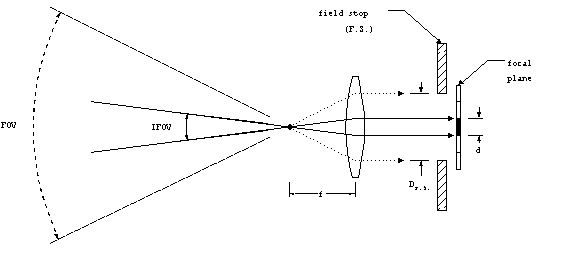Figure 2. Fields-of-view.

As can be seen from the geometrical construction in figure 2, the diameter of the field stop, DF.S. affects the FOV. If the field stop is made smaller, the FOV will be reduced accordingly. By analogous reasoning, the instantaneous field-of-view (IFOV) will be affected by the size of the individual detecting element, d. The IFOV is the range of incident angles seen by a single detecting element in the focal plane.

The IFOV and FOV can be calculated using trigonometry: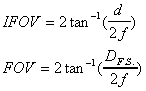Equation 1Equation 2

For small angles, less than 200, which is generally true for IFOV, the inverse tangent can be accurately approximated by tan-1(x) x (radians), in which case:

IFOV d/f, (if d/f <<1).

Equation 3

Example: digital camera. Find the FOV and IFOV for a 35 mm digital camera with a 50 mm lens, that uses 1152 x 864 resolution.

First, "decode" the terminology:

50 mm lens focal length f = 50 mm.

35 mm the field stop DF.S. = 35 mm (for a conventional film camera, this is the size of the film).

1152 x 864 resolution there are 1152 pixels in the horizontal direction and 864 in the vertical.

Therefore, the FOV is calculated using equation (1):

FOV = 2 tan-1(35/2x50) = 38.50

The instantaneous field-of-views, either from equation (2), or by noting the FOV must be divided by the number of elements to get the IFOV:

HIFOV (horizontal) = FOV/1152 = 0.030
VIFOV (vertical) = FOV/864 = 0.0450

Depth of Focus

The focal plane must be placed so that objects at the desired range will be in focus. For any object range, all the rays from that object will come together at a unique location beyond the lens. If the object is at a very long range, the rays will come together at the focal point. At shorter ranges, that point gets further and further from the lens.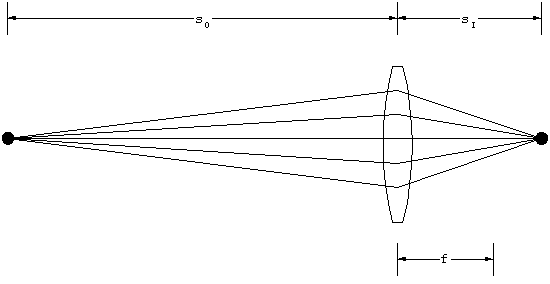Figure 3. Object and image distances.

The distance of the object in front of the lens, sO, and the location of the focused image behind the lens, sI, are related by the Lens Maker's Equation

1/sO + 1/sI = 1/f.

Equation 4

If the focal plane is so located that the object is in perfect focus, meaning the object and image distances satisfy equation (4), the question becomes: at what range from the object does the image become noticeably unfocused? As the object distance is changed, its image will become spread out on the focal plane. When the image becomes so spread out that it overlaps the adjacent detecting elements, the overall image will be distorted. So, the size of the detecting element determines the limits beyond which the image is considered to be unfocused. The range of object distances which satisfy the criterion for making a suitably focused image is called the depth of focus.

The depth of focus depends initially on the detecting element size and the focal length. In practice the depth of focused can be controlled. This is accomplished by the use of an aperture stop (A.S.). As we have already seen the aperture stop will limit the amount of flux that is collected. So it would seem that the largest possible aperture stop is the most beneficial. However, the larger the aperture stop, the shorter the depth of focus. This is illustrated by another geometrical construction.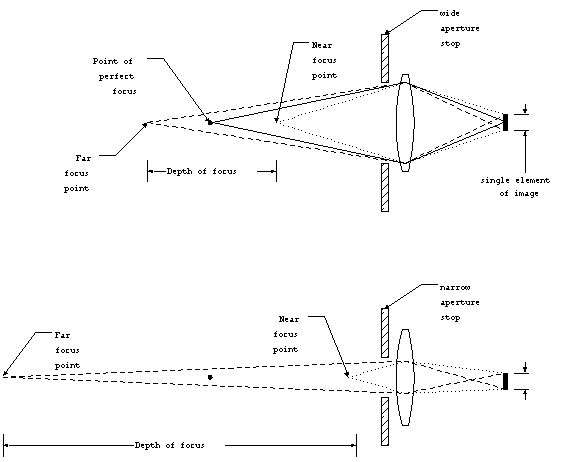Figure 4. Depth of focus.

By limiting the range of angles at which the rays may enter the optics, the aperture stop actually improves the depth of focus. As a trade-off, however, reducing the aperture stop limits the amount of flux that can be collected by the detecting system, and therefore would require more sensitive detecting elements to achieve the same maximum detection range. This is why smaller apertures require longer exposure times in conventional photography.

Scanning vs. Staring Sensors

In the previous discussion, it was assumed that at the focal plane, there was an array of detecting elements, one for each part of the image within the field-of-view. This configuration is used in staring sensors. The resolution of the object within the field of view is determined by the IFOV, which can be found from equations (2) or (3).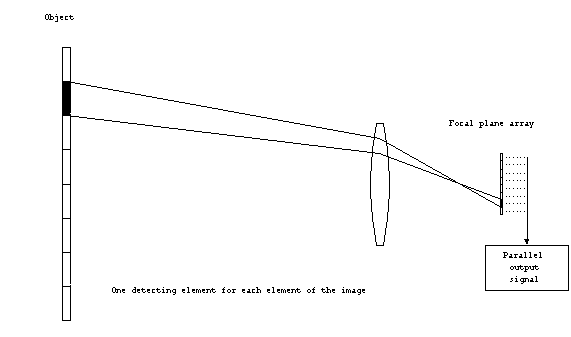Figure 5. Staring (parallel
scan) system.

All of the detecting elements are simultaneously exposed to the image from the object, and therefore can produce output in parallel. The output of the detecting elements is scanned once to create a complete image, or frame. In standard video, each frame lasts 1/30 of a second. The frame rate is how often the frame is changed, therefore, the frame rate would be 30 Hz. The frame rate limits how long each element of the image is incident upon the detecting element, known as the dwell time, tdwell. In a staring system, the dwell time is the same as the duration of the frame, or the reciprocal of the frame rate. Generally, the longer the dwell time, the more sensitive the detector is. Alternately, the longer dwell time reduces the electrical bandwidth and therefore the noise of the detector, which has the same result.

A single detecting element can be used in a scanning system. In this configuration, some device is used to sequentially scan the instantaneous field-of-view (here determined by the aperture) to a single detector. The scanning system might be a rotating mirror which directs the IFOV onto a single element. The scanning system might be used in applications where the detecting elements are very expensive. Since only a single element is used, it is much cheaper than the typical, 640x400 array of a staring sensor.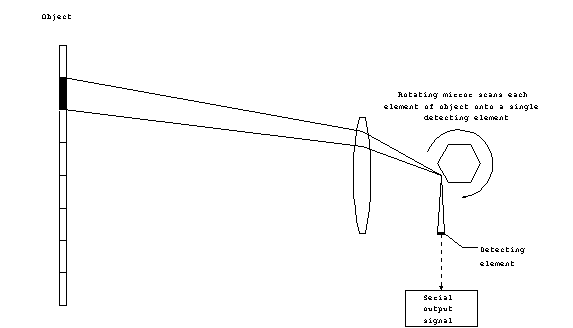Figure 6. Scanning (serial
scan) system.

Since only the IFOV is directed on the detector at any one time, the output of the scanning system is serial. For a scanning system, the dwell time is determined not only by the frame rate, but also by the total number of elements (or pixels) in one complete image. For example, the 640x400 pixel image of the standard VGA monitor uses 1/256,000 the dwell time as compared to an equivalent staring system. The reduced dwell time increases the electrical bandwidth (recall the BW 1/2t relationship), which can in turn increase the noise in the system.

Resolution

Spatial Resolution

The spatial resolution of an imaging system is its ability to distinguish separate objects or parts of an object within its field-of-view. The smallest image element is determined by the IFOV. There may be different vertical (VIFOV) and horizontal (HIFOV) instantaneous fields-of-view. For an object at some range, R, from the detector, the IFOV will encompass some length. For example, if the IFOV = 1 mrad, then at 1000 m, the instantaneous field-of-view will cover 1 m in length. The spatial extent of the IFOV at object range R is estimated (for small angles) by

height: Dh R x VIFOV

width: Dw R x HIFOV

Equation
5Equation 6

Example: find the spatial resolution at 500m of a staring sensor with a FOV = 100 x 100 using a 100 x 100 detecting element focal plane array.

Since the FOV in either direction is 100, the IFOV is just 1/100 th or:

HIFOV= VIFOV = 0.10 = 1.7 mrad

At 500 m,

Dh = Dw = 500 m (0.0017) = 85 cm

Thermal Resolution

For infrared imaging systems, which detect the thermal radiation from objects, an important measure of performance is its ability to detect small changes in temperature. The smallest temperature difference a system can detect (and therefore can display differently) is called the thermal resolution. Changes which are too small to be distinguished from the background noise in the system will not be detected. Sometimes thermal resolution is described by NETD, which stands for noise-equivalent-temperature-difference. NETD is the temperature change which changes the collected flux by an amount equal to the noise-equivalent-power (NEP).

The thermal resolution (or NETD) can be improved by increasing the size of the detecting elements, since more flux will be collected by each. Unfortunately, this would degrade the spatial resolution, by increasing the IFOV. As a general result (which is not proven here) the thermal and spatial resolution are inversely proportional.

Spatial and Thermal Resolution, MRTD

Since it is not possible to simultaneously achieve high spatial and thermal resolution, neither is a good measure of the overall IR imaging system performance. A single quantity, called the minimum resolvable temperature difference, MRTD, measures both performance factors simultaneously. MRTD is determined experimentally and therefore takes into account all of the various theoretical and real-world factors that matter. The measurement is done by slowly heating a test pattern at some range from the detector. The target is shown below: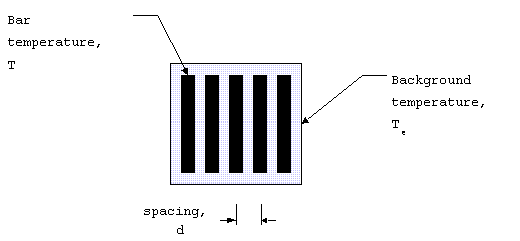Figure 7. MRTD target.

From one bar to another is a single cycle of the test pattern (like waves). Since the spacing is d, the spatial frequency is 1/d with units of cycles/m. Since the spatial extent is related to the IFOV by the range, the spatial frequency can be expressed as cycles/mrad calculated from 1000/(R d).

MRTD is the temperature difference at which bars first becomes visible against the background. MRTD has units of oC at at a given spatial frequency (in cycles/mrad). MRTD combines both spatial and thermal resolution into a single quantity that can be used to compare systems.

Example: MRTD = 0.05oC at 0.5 cycles/mrad,

compute the thermal and spatial resolution at 1000 m.

The thermal resolution is 0.05o, which represents that smallest temperature change that can be detected, at any range.

The spatial resolution can be calculated from equations (5) and (6). First compute the IFOV:

Therefore at R = 1000m, the spatial resolution is
Dw = R x IFOV = (1000 m) x (0.002)

Dw = 2m.

Infrared Search and Tracking (IRST) Systems

A passive infrared tracking system has the additional complication of range determination. Unlike radar, which can measure the range directly, the passive system must use other means. There are two main ways in which range information is obtained: triangulation and in combination with a laser range-finder.

Triangulation (passive)

This requires two or more sensors, preferably very far apart. The accuracy of the system improves with the separation distance. What is required is a difference in measured bearing, Dq, to the target from the two sensors which are separated by distance d.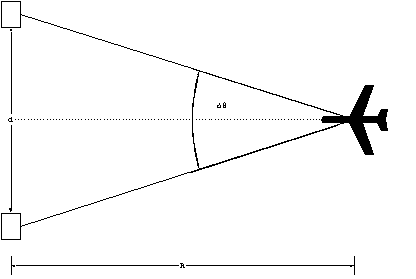Figure 8. Triangulation.

If the sensors are spaced along a line perpendicular to the direction of the target, the range is determined by:

R = d/2tan(Dq/2)

Equation 7

The limit of the system is reached when the difference in bearing is equal to the HIFOV, therefore the maximum range at which the system can function is

Rmax = d/2tan(HIFOV/2)

Equation 8

Example: suppose the HIFOV is 1 mrad (0.06o) and the sensors are spaced by 10 m, find the maximum triangulation range.

Rmax = 10/2tan(0.06o/2) = 9500 m.

Laser range-finder (passive-active combination)

The laser operates in a pulsed mode, and obtains the range in an identical manner to a pulsed radar system. This can be used for continuous range tracking when combined with the bearing and elevation tracking from the IR system. Since the laser beam has a very small beamwidth, it is necessary to use the bearing and elevation tracking to aim the laser at the target. The laser range finder will have the parameters of PW and PRF just like radar, with the same implications. For example, minimum and maximum unambiguous range. However, the pulsed laser range finder is generally unsuitable for Doppler measurement because the first blind speed is very low (order of cm/s).

Visible Band Imaging Systems

Thermal radiators at reasonable temperatures all emit energy in the infrared band. In order for the radiation to be in the visible band requires temperatures akin to the surface of the sun. Therefore, detectors that operate in the visible band (0.4 to 0.7 mm) cannot be used to detect thermal radiation. They can, however, improve upon normal human eyesight. There are two main things that visible imaging systems can improve upon: magnification and light amplification.

Magnification

We are all undoubtedly familiar with magnifying system like binoculars and telescopes. These can also be thought of as parts of weapons systems. For example, the scope on a rifle, or the periscope on a submarine. In fact, these can provide highly accurate bearing, elevation and range which is all you could ask of any weapons sensor.

The magnification for a simple thin lens can be derived by the following construction: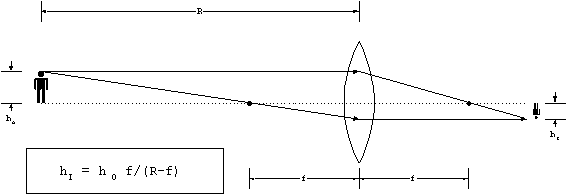Figure 9. Transverse magnification.

For relatively long ranges, where R >> f, this simplifies to hI = hO f/R. The transverse magnification is the ratio of the image to the object:

MT hI/hO f/R, for R>>f

Equation 9

To make a telescopic sight, or scope, two lenses are combined: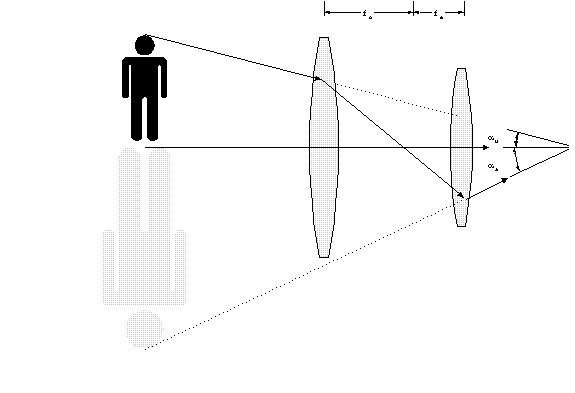Figure 10. Angular magnification.

If the object subtends an unaided viewing angle, au, then the combination of lenses alters the incoming rays so that they appear to subtend an aided viewing angle, aa. When aa is larger than au, the object appears larger and is therefore magnified. The angular magnifying power, MP, is defined by

MP aa/ au

Equation 10

The correct combination is achieved by placing the focal point of the objective lens at the focal point of an eyepiece lens. When this is done, the relationship between the focal lengths of the objective and eyepiece lens will determine the magnification power. If we consider rays coming in from a great distance, the relationship can be constructed: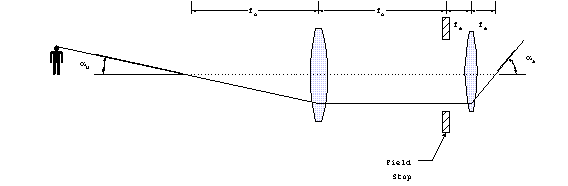Figure 11. Magnifying
power.

The magnifying power is found from

MP = aa/ au = tan-1(DF.S. /2fo)/tan-1(DF.S./2fe)

As long as we are only considering small viewing angles (unlike the illustration), which is normally the case the magnification power is

MP fo/fe

If the aperture stop of the objective optics is Do, the image size of the objective after it passes through the system will be De. Therefore

MP = fo/fe = Do/De

Equation 11

Binoculars and telescopes are specified by the two-number combination: MP x Do (in mm). For example, 7x50 binoculars have MP = 7 and Do = 50 mm. The larger the objective diameter, the better the performance in low light situations. The field-of-view is controlled by the field stop. Generally binoculars have a set field-of-view which is about 80. You may also have noted that this simple system would result in an inverted image. In practice, this is corrected by an erecting system between the objective and eyepiece lenses.

Range can be obtained from a strictly passive visible-light optical system only if the size of the object is known. The principle if based on trigonometry (again!). Suppose we have and object with height, h, at some unknown range, R. If the angular extent of the object is measured to be q, then the range can be determined from

R = h/tan(q)

Equation 12

Of course, this result is not particularly handy since it would require a calculator. This result is commonly used when the angle is small, for example 10. If we express the angle in radians, then the small angle approximation can be used,

tan(q) q

which converts the stadimeter range equation into

or more if the angle is in degrees, then

R 60h/q, angle in degrees.

An object 100 feet tall will subtend 1o at 1 nautical mile

This can be used to derive the handy thumbrule:

For other heights or angles the range can be found by taking a ratio.

Example: Using binoculars with an 8o field-of-view, you observe a 1200 ft. tower which fills one-quarter of the FOV. Find the range to the tower.

The angle is 20, which is one-quarter of 8o.

If a 100 ft. tower subtends 1o at 1 nm, then it would subtend 20 at 0.5 nm.

The 1200 ft, tower subtends 20 at 12 x 0.5 nm, or 6 nm.

R = 6 nm.

Another example: periscope ranging.

Most periscopes have an 8o field-of-view in high-power. The optics has 32 vertical marks used for ranging. Each division is 1/40 and therefore, the typical ship with a masthead at 100 ft, will subtend one division when it is at 4 nm.

Light Amplification

So called low-level-light (LLL) systems are designed to enhance the light which is reflected from targets. Unlike infrared, LLL systems require some background illumination in order to function. These systems only amplify what is already there from external sources like moon or star light. The output from the eyepiece of an ordinary scope is put into an image intensifying tube. This consists of three parts:

Photocathode. The incoming light causes the photocathode to give off electrons.

Electrodes. These are pairs of plates at a high voltage difference, so that the electrons are accelerated between them. The electrons move from the cathode to an anode. In order to achieve high gain, in between are placed dynodes, which act as both anode and cathode. The electrons are collected from the photocathode at the first dynode. This causes the emission of secondary electrons, which in turn are accelerated again. Each pair of dynodes acts as a stage, and at each stage, the total number of electrons in increased.

Phosphorus plate. After the number of electrons has been increased many times, a phosphorus plate is placed just at the other end from the photocathode. When the electrons strike the plate, it emits light. Typically the light is green. Gain is achieved by increasing the number of electrons in stages. Using many stages, the light can be increased by over 30,000 times what came into the detector.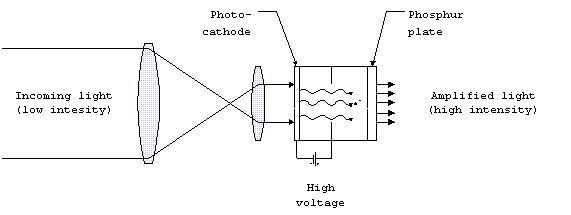Figure 12. Image intensifying
system.

LLL systems intensify the amount of light already present. They will not work if there is no light present. Additionally, they can become saturated if too much light is present.

The resolution of the LLL system is determined by the size and number of detecting elements. As a general rule, the resolution varies inversely with the sensitivity. For example, using many stages, the number of electrons reaching the phosphorus plate can be increased greatly. When the electrons reach the plate, it will be difficult to finely focus this hoard of electrons back into the small elements of the image without any overlap or interference. Another way to improve sensitivity would be to collect more light at the entrance (objective lens) by increasing the aperture stop. This, however, would affect the depth of focus.

Low-level-light systems are not suitable for precise imaging by the very nature of their operation, so it is not a big deal if the image quality is low. What is needed is the detection of the presence of objects such as troops, or vehicles.

LLL systems may be combined with their own light sources for use in conditions where no ambient light exists, for instance inside of buildings. Of course, the system is no longer passive and its use can be detected. Furthermore, LLL systems can be combined with narrow beamwidth laser light sources, which can produce a bright reflection off the target in order to provide accurate weapons sighting.

Laser Target Illumination

Since laser light has a narrow beamwidth it is well-suited to precise measurement. A laser tracking system, using the same design as the radar servo tracking system, would be extremely accurate. As an added benefit, the reflected beam can be used to guide weapons into the target. When used in this manner, the laser servo tracking system is called an target illuminator. Target illumination can be provided by either the weapon itself, or more commonly, by a third party.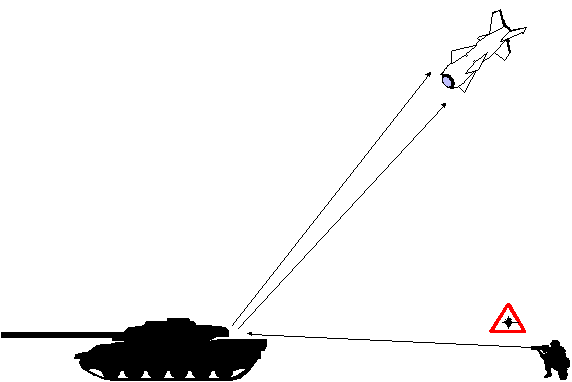Figure 13. Target illumination.